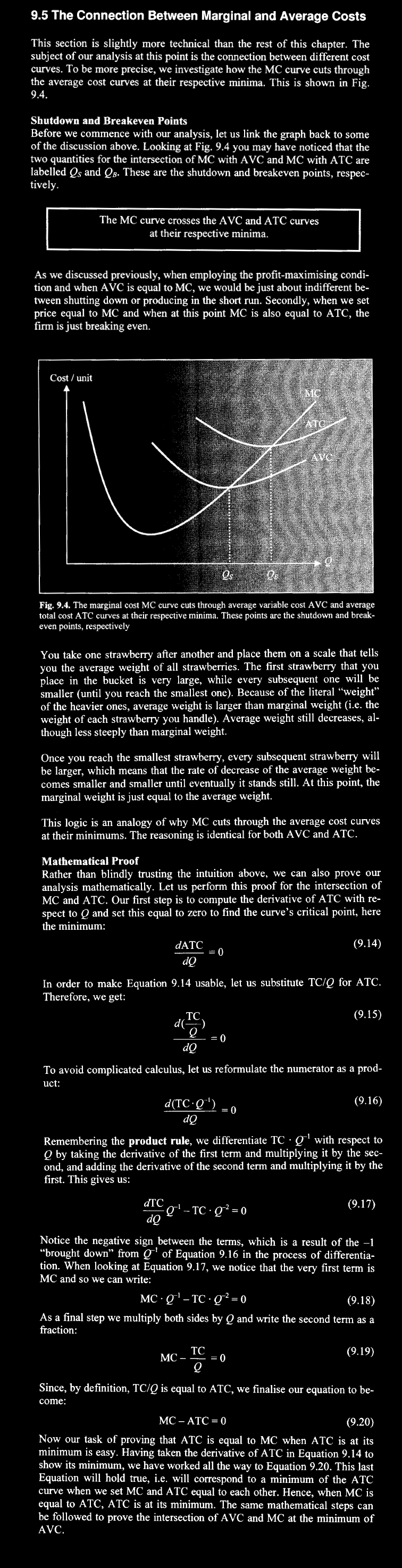# The strawberry theorem in economicsLecture on economics we proved the Strawberry theorem:
```If f is a function and F its antiderivative and g(x)=F(x)/x then
the graphs of f and g intersect at critical points of g.
```
Despite the fact that the proof is a simple computation:
```   g'=(F(x)/x)' = F'/x - F/x^2 = (1/x) (F'-F/x) = (1/x) (f-g)  ,
```
mathematicians can find this theorem intriguing per se. The theorem really comes to life in economics: if F is the total cost, then f the marginal cost and g the average cost. Quantities x for which f=g are called break even points. The theorem tells that break even points usually happen at extrema of g. If F is concave up, break even points are minima. I chose the name Strawberry theorem because of the following beautiful story I had found in the book by "Dominik Heckner and Tobias Kretschmer with the title Don't worry about Micro.

 Suppose you have all sizes of strawberries, from very large to very small. Each size of strawberry exists twice except for the smallest, of which you only have one. Let us also say that you line these strawberries up from very large to very small, then to very large again. You take one strawberry after another and place them on a scale that sells you the average weight of all strawberries. The first strawberry that you place in the bucket is very large, while every subsequent one will be smaller until you reach the smallest one. Because of the literal weight of the heavier ones, average weight is larger than marginal weight. Average weight still decreases, although less steeply than marginal weight. Once you reach the smallest strawberry, every subsequent strawberry will be larger which means that the rate of decease of the average weight becomes smaller and smaller until eventually, it stands still. At this point the marginal weight is just equal to the average weight

Here is a scan from the book.